## Homework Problems

1. Find the allowed total spin states of two spin 1 particles. Explicitly write out the 9 states which are eigenfunctions of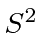and.

2. The Hamiltonian of a spin system is given by. Find the eigenvalues and eigenfunctions of the system of two particles (a) when both particles have spin, (b) when one particle has spinand the other spin 1. What happens in (a) when the two particles are identical?

3. Consider a system of two spinless identical particles. Show that the orbital angular momentum of their relative motion can only be even.Show by direct calculation that, for the triplet spin states of two spinparticles,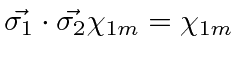for all allowed. Show that for the singlet state.

4. A deuteron has spin 1. What are the possible spin and total angular momentum states of two deuterons. Include orbital angular momentum and assume the two particles are identical.

5. The state of an electron is given by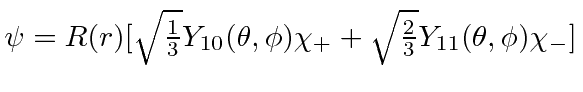. Find the possible values and the probabilities of the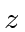component of the electron's total angular momentum. Do the same for the total angular momentum squared. What is the probability density for finding an electron with spin up at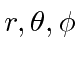? What is it for spin down? What is the probability density independent of spin? (Do not leave your answer in terms of spherical harmonics.)

6. Thestates of hydrogen have an 8-fold degeneracy due to the variousandstates allowed and the two spin states of the electron. The spin orbit interaction partially breaks the degeneracy by adding a term to the Hamiltonian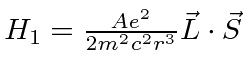. Use first order perturbation theory to find how the degeneracy is broken under the full Hamiltonian and write the approximate energy eigenstates in terms of,, and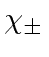.

7. The nucleus of a deuterium (A=2 isotope of H) atom is found to have spin 1. With a neutral atom, we have three angular momenta to add, the nuclear spin, the electron spin, and the orbital angular momentum. Define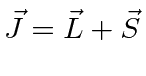in the usual way andwhere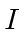denotes the nuclear spin operator. What are the possible quantum numbersandfor an atom in the ground state? What are the possible quantum numbers for an atom in the 2p state?

Jim Branson 2013-04-22